## Numbers To Roman Numerals Converter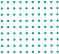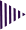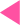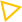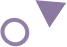Best Roman Numeral Converter calculator

# Roman Numeral Converter

In Roman Numerals, a number consists of the symbols (or letters/characters) C, D, I, L, M, V, X written in a specific order. We see Roman numerals everywhere - clocks, school classes, musical chords, historical dates, legal documents, astronomy, and even cricket team names. Based on the ancient Roman system, Roman numerals are the symbols used in numerical notation. Check out the table below to see how they correlate.
In these numerals, letters are used together to represent numbers. An example of a roman numeral is XLVIII which is equivalent to 48 in numeric form. The Roman numerals use a combination of symbols, including the English alphabet. As an example, 5 in roman numeral is denoted by V. It may be used for anything, including Class I, Class II, Class X, etc.
In Roman Numerals, a number consists of the symbols (or letters/characters) C, D, I, L, M, V, X written in a specific order.
1.) An "I" before an "V" or "X" represents one less, so four is IV (one less than 5), and nine is IX (one less than 10) if "I" comes before an "V" or "X".
2.) The "X" before L or C represents ten less, so 40 is XL (10 less than 50), and 90 is XC (10 less than 100).
3.) In the case of "C" placed before D or M, it represents a hundred less, so 400 is CD (100 less than 500), and 900 is CM (100 less than 1000).
Enter the number you'd like to convert to Roman numerals. Because Roman numerals can't represent fractions, the number zero, negative numbers, or numbers above3,999,999, the Roman numerals generator will reject such inputs.

## Roman Numerals Calculation

 Roman Numeral Calculation to get the number Roman Numeral Calculation to get the number I 1 = 1 XVI 10 + 5 + 1 = 16 II 1 + 1 = 2 XXVII 10 + 10 + 5 + 1 + 1 = 27 III 1 + 1 + 1 = 3 XXXVIII 10 + 10 + 10 + 5 + 1 + 1 + 1 = 38 IV 5 – 1 = 4 XXXIX 10 + 10 + 10 – 1 + 10 = 39 V 5 XL -10 + 50 = 40 VI 5 + 1 = 6 XLI -10 + 50 + 1 = 41 VII 5 + 1 + 1 = 7 XLII -10 + 50 + 1 + 1 = 42 VIII 5 + 1 + 1 + 1 = 8 XLIII -10 + 50 + 1 + 1 + 1 = 43 IX 10 – 1 = 9 XLIV -10 + 50 – 1 + 5 = 44 X 10 XLV -10 + 50 + 5 = 45 XI 10 + 1 = 11 XLVI -10 + 50 + 5 + 1 = 46 XII 10 + 1 + 1 = 12 XLVII -10 + 50 + 5 + 5 + 1 = 47 XIII 10 + 1 + 1 + 1 = 13 XLVIII -10 + 50 + 5 + 1 + 1 + 1 = 48 XIV 10 – 1 + 5 = 14 XLIX -10 + 50 – 1 + 10 = 49 XV 10 + 5 = 15 L 50 XVI 10 + 5 + 1 = 16 LX 50 + 10 = 60 XVII 10 + 5 + 1 + 1 = 17 LXX 50 + 10 + 10 = 70 XVIII 10 + 5 + 1 + 1 + 1 = 18 LXXX 50 + 10 + 10 + 10 = 80 XIX 10 – 1 + 10 = 19 XC -10 + 100 = 90 XX 10 + 10 = 20 C 100 XXI 10 + 10 + 1 = 21 CC 100 + 100 = 200 XXII 10 + 10 + 1 + 1 = 22 CCC 100 + 100 + 100 = 300 XXIII 10 + 10 + 1 + 1 + 1 = 23 CD -100 + 500 = 400 XXIV 10 + 10 – 1 + 5 = 24 D 500 XXV 10 + 10 + 5 = 25 DC 500 + 100 = 600 XXVI 10 + 10 + 5 + 1 = 26 DCC 500 + 100 + 100 = 700 XXVII 10 + 10 + 5 + 1 + 1 = 27 DCCC 500 + 100 + 100 + 100 = 800 XXVIII 10 + 10 + 5 + 1 + 1 + 1 = 28 CM -100 + 1000 = 900 XXIX 10 + 10 – 1 + 10 = 29 M 1000 XXX 10 + 10 + 10 = 30 ¯¯¯¯VV¯ 5000 XXXI 10 + 10 + 10 + 1 = 31 ¯¯¯¯¯XX¯ 10000 XXXII 10 + 10 + 10 + 1 + 1 = 32 ¯¯¯¯LL¯ 50000 XXXIII 10 + 10 + 10 + 1 + 1 + 1 = 33 ¯¯¯¯CC¯ 100000 XXXIV 10 + 10 + 10 – 1 + 5 = 34 ¯¯¯¯¯DD¯ 500000 XXXV 10 + 10 + 10 + 5 = 35 ¯¯¯¯¯¯MM¯ 1000000